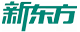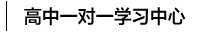#400-817-1616

## 数学线性公式总结

来源：网络

时间： 2018-11-16 16:30

抛物线：y=ax*+bx+c

就是y等于ax的平方加上bx再加上c

a>0时开口向上

a<0时开口向下

c=0时抛物线经过原点

b=0时抛物线对称轴为y轴

还有顶点式y=a(x+h)*+k

就是y等于a乘以(x+h)的平方+k

-h是顶点坐标的x

k是顶点坐标的y

一般用于求最大值与最小值

抛物线标准方程：y^2=2px

它表示抛物线的焦点在x的正半轴上，焦点坐标为(p/2，0)准线方程为x=-p/2

由于抛物线的焦点可在任意半轴，故共有标准方程y^2=2pxy^2=-2pxx^2=2pyx^2=-2py

关于圆的公式

体积=4/3(pi)(r^3)

面积=(pi)(r^2)

周长=2(pi)r

圆的标准方程(x-a)2+(y-b)2=r2注：(a，b)是圆心坐标

圆的一般方程x2+y2+Dx+Ey+F=0注：D2+E2-4F>0

(一)椭圆周长计算公式

椭圆周长公式：L=2πb+4(a-b)

椭圆周长定理：椭圆的周长等于该椭圆短半轴长为半径的圆周长(2πb)加上四倍的该椭圆长半轴长(a)与短半轴长(b)的差。

(二)椭圆面积计算公式

椭圆面积公式：S=πab

椭圆面积定理：椭圆的面积等于圆周率(π)乘该椭圆长半轴长(a)与短半轴长(b)的乘积。

以上椭圆周长、面积公式中虽然没有出现椭圆周率T，但这两个公式都是通过椭圆周率T推导演变而来。常数为体，公式为用。

椭圆形物体体积计算公式椭圆的长半径*短半径*PAI*高

（责任编辑：刘汉甜）